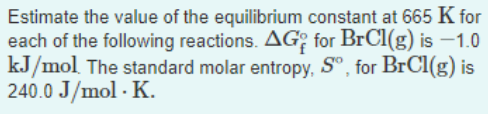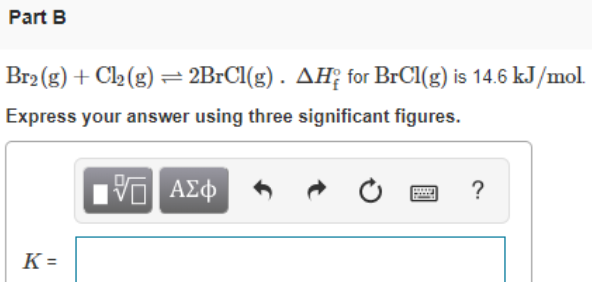# Estimate the value of the equilibrium constant at 665 K for each of the following reactions. ΔG°f for BrCl(g) is -1.0 kJ/mol. The standard molar entropy, S°, for BrCl(g) is 240.0 J/mol.K. Br2(g) + Cl2(g) ⇌ 2BrCl(g). ΔH°f for BrCl(g) is 14.6 kJ/mol Express your answer using three significant figures.